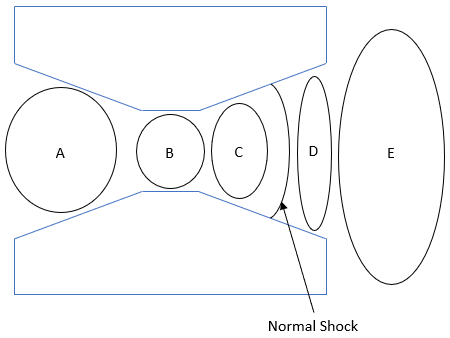## Mach Number

The below figure is a cross section of a testing chamber with a converging/diverging nozzle. If a gas mixture (c = 300 m/s) experiences a normal shock wave as shown by being accelerated from 250 m/s through the chamber’s nozzle, what section is the Mach number = 1?Hint
The Mach Number is a dimensionless value useful for analyzing fluid flow dynamics problems where compressibility is a significant factor.
$$Ma=\frac{v}{c}$$$where $$Ma$$ is the Mach number, $$v$$ is the fluid flow speed, and $$c$$ is the speed of sound. Hint 2 In a normal shock situation, flow slows down from supersonic to subsonic, so section C has $$Ma>1$$ and section D has $$Ma< 1$$ . The Mach Number is a dimensionless value useful for analyzing fluid flow dynamics problems where compressibility is a significant factor. $$Ma=\frac{v}{c}$$$
where $$Ma$$ is the Mach number, $$v$$ is the fluid flow speed, and $$c$$ is the speed of sound (which varies based on medium conditions).
• If the mach number is  < 1 , the flow speed is lower than the speed of sound - and the speed is  subsonic .
• If the mach number is  ~ 1 , the flow speed is approximately like the speed of sound - and the speed is  transonic .
• If the mach number is  > 1 , the flow speed is higher than the speed of sound - and the speed is  supersonic .
• If the mach number is  >> 1 , the flow speed is much higher than the speed of sound - and the speed is  hypersonic .

The flow is subsonic in the chamber because $$Ma=250/300=0.83$$ , so $$Ma< 1$$ in section A. Flow in a converging/diverging nozzle transitioning from subsonic to supersonic will have $$Ma=1$$ at the throat, so section B is the transonic phase. In a normal shock situation, flow slows down from supersonic to subsonic, so section C has $$Ma>1$$ and section D $$Ma< 1$$ . Because section E expands to an ambient environment, the flow must be subsonic.
B